Disclosure: This post may contain affiliate links, meaning when you click the links and make a purchase, we receive a commission.

# Count Cells that Contain Specific Text in Excel

While working with a big range of data it is often required to find the number of specific text. In this article, we will count cells that contain specific text. In Excel, counting cells that contain specific text is an important task. We will also count cells that contain any text.

## Count Cells that Contain Text

Before counting cells that contain specific text we should see now the process of counting cells which contain any text. Two formulas can be used for counting cells that contain text. Here we have a list which ranges from A1 to B12. A1 and B1 cells contain the headings. We will be calculating cells that contain text for the range A2: B12. Write down the below formulas in any blank cells of your worksheet to find the result.

Formula (1) `=COUNTIF(A2:B12,"*")`

Formula (2) `=SUMPRODUCT(--(ISTEXT(A2:B12)))`

After applying the above two formulas you will get to see the below result.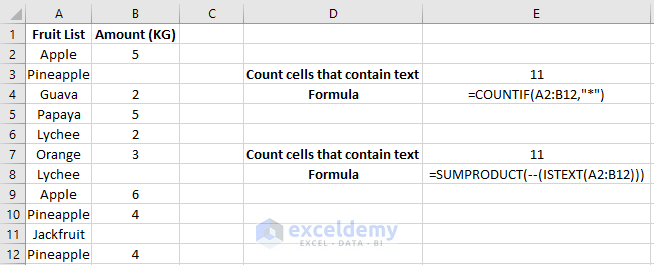## Count Cells That Contain Specific Text

To count cells that contain specific text we will be using the same formula with a different argument. Let`s say we want to find the number of cells that have apples in it. It can be done in two ways. One process is finding the cells which contain the text apple (both apple & pineapple) anywhere in a text. Another process is finding the number of cells that contain the text “Apple” only.

### Count cells with Match Criterion

For counting cells with match criterion type the formula, `=COUNTIF(A2:A12,"*Apple*")` anywhere in your worksheet. Here in this worksheet, we placed it in cell E3. You can also indicate cell instead of text for finding the same result. Look at the below picture to get a clear view of the formulas and the results.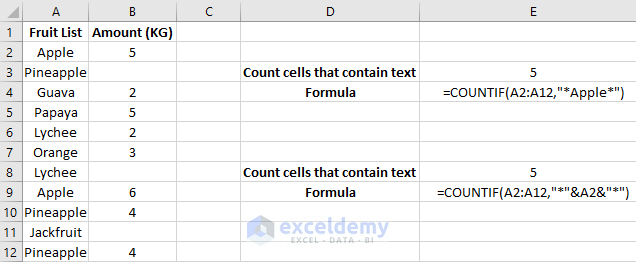The formulas count all the cell that has the text apple in the range A2: A12.

### Matching Specifically One Text

To count cells that have only the text “apple” we can use the formula`=COUNTIF(A1:A10,"Apple").` In cell E3, of the below picture we used this formula and found out there are exactly two cells that contain the text apple only. You can either write the cell name or specify the cell name to find the result. Like, instead of writing “apple” in the formula you can also write only A1 as it contains the text Apple.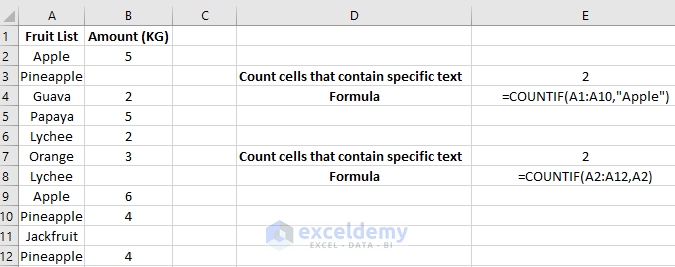Let`s make this more dynamic by indicating the fruits name in a different cell. You can use the data validation. Here we make a list using the data validation in cell E7 so that we can insert any fruit to count them in the list. The formula is applied in cell E8.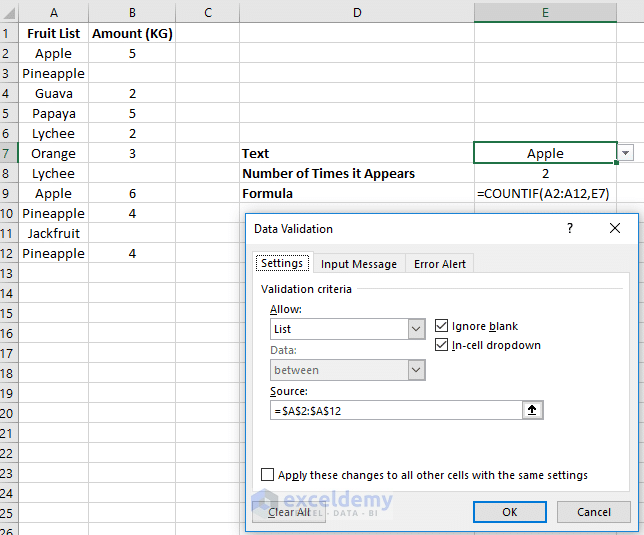After applying data validation you will get the below result.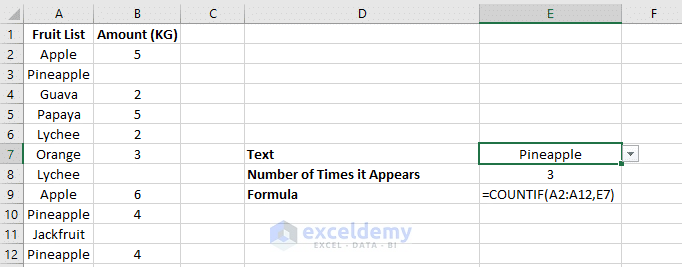## Count Cells that Contain Specific One Text with a Different Formula

We can use a different formula to count cells that contain a specific text. The formula has 3 functions which are, SUMPRODUCT, ISNUMBER and FIND. You can use the formula `=SUMPRODUCT(--(ISNUMBER(FIND("Apple",A2:A12))))` or `=SUMPRODUCT(--(ISNUMBER(FIND(A2,A2:A12))))` for this. The formulas along with the result are shown below.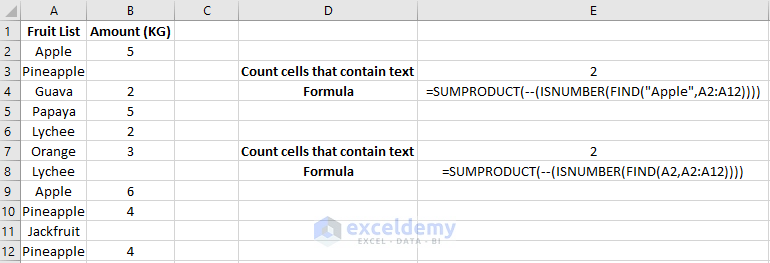## Count Cells with Multiple Substring

Let`s say you want to calculate the number of cells which full fill more that one text criterion. In this example, we have a list where different items are listed along with its color and size. We want to count the item T-Shirt with color blue which is in the range of A2: A9. To do this write down the formula `=COUNTIFS(A2:A9,"*T-Shirt*", A2:A9,"*Blue*")` or

`=COUNTIFS(A2:A9,"*"&D2&"*", A2:A9,"*"&D3&"*") `in cell D4. You will find the accurate result that you want.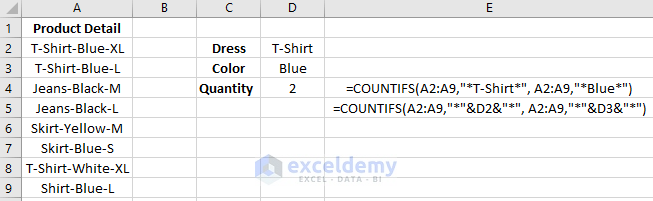## Excel VBA to Count Cells that Contain Specific Text

We can use Excel VBA code for counting cells of specific text. To perform this, click on the Visual Basic under the Developer tab and insert the below code in there.

`Sub COUNT_TEXT()`

`Dim iVal As Integer`

`iVal = Application.WorksheetFunction.CountIf(Range("A2:A12"), "Apple")`

`MsgBox iVal`

`End Sub`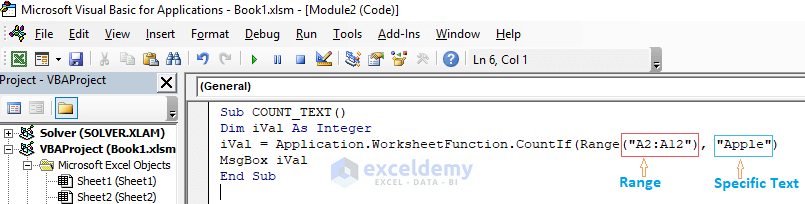Here you can edit the range and specific text to perform in your worksheet. After running the code, you will get the below result.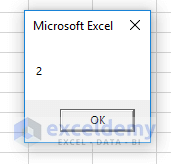You can use the below code if you want to count your specific text row-wise. Insert the below code in your VBA module and run it.

`Sub blah()`

`For j = 1 To 12  'Adjust Rows`

`  count = 0`

`  For i = 1 To 2 Step 12 'Adjust Columns`

`    If Cells(j, i) = "Apple" Then count = count + 1`

`  Next i`

`  MsgBox "Count of 'Apple's in row " & j & " is " & vbLf & count`

`Next j`

`End Sub`

After running the code, you will see that a text box appears which will tell you the number of time your text appears in the 1st row. After pressing Ok, it will tell you the number of appearance of that specific text in the 2nd row. It will continue to appear in total 12 times.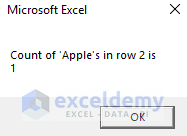## Related Articles

Hello!

Welcome to my Profile. Here I will be posting articles related to Microsoft Excel. I have completed my BSc in Electrical and Electronic Engineering from American International University-Bangladesh. I am a diligent, goal-oriented engineer with an immense thirst for knowledge and attitude to grow continuously. Continuous improvement and life-long learning is my motto.

1. ReplyDavid Baker August 9, 2018 at 8:33 PM

very intresting account always thought there was a way to handle the need. If I can here’s a question I have tried to have the sheet highlight data in cells but every time I it doesn’t work out. Can you possibly cover that if you haven’t yet. Thanks in advance

2. ReplyGreg Beardshaw January 9, 2019 at 9:00 PM

I was able to add conditional formatting by using the same formula. Where A2 used a formula to determine which cells to format, and the formula → =COUNTIF(A3:A3,”*Apple*”)=1
Then copied this to the column.

• ReplyJanuary 10, 2019 at 3:50 PM